-10%

# Primary Math kit

Rs. 4,041.00 Rs. 3,637.00

In StockIn StockOut of stock

Shop more, SAVE more!

🚚 Cash On Delivery (COD) available

⚒️ Broken/ lost parts? Get free replacements

🔙 10 day returns

💥 Shop above Rs 2000 & get Rs 10% OFF, apply code: GET10

📦 Free Shipping on all orders above Rs. 500

💳 10% OFF on Prepaid Orders max Rs. 500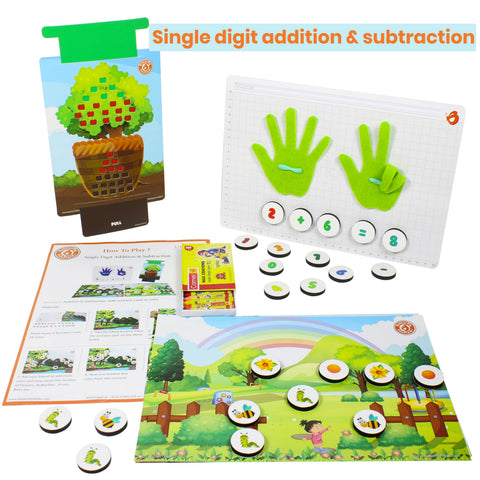Learn Counting with Hands (Finger Counting), Single digit Addition & Subtraction using Magnetic pieces, Slider board & Finger Counter.Place Value - 2, 3 & 4 Digit Numbers | Math

* Use blocks and tiles to understand the need for place value

* Learn expanded form of representing numbers using the place value strips (1-9, 10-90, 100-900, 1000-9000)

* Compare 4 digit numbers using the place value strips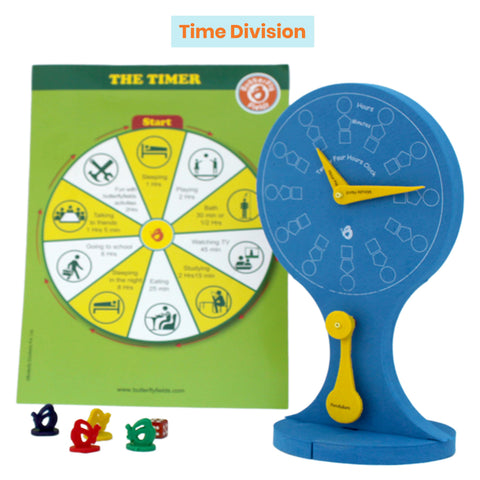Time Division

* Make a clock and learn to tell the time.

* Identify the hour-hand and the minute-hand.

* Learn the difference between 12-hour and 24-hour format.

* Learn how much work and play can be done in 24 hours by playing a game.Different Shapes | Basics of Geometry | Math

• Make different shapes using sticks and clay to understand the
difference between lines and joining points.

• Solve a jig-saw puzzle using different shapes based on their no. of
sides & joining points

• Learn to guess the shape of an object from its shadow.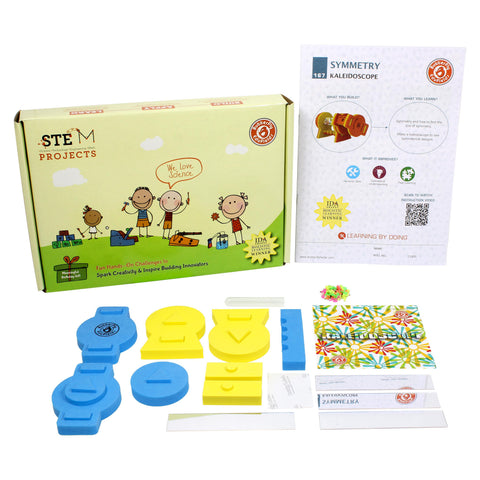Identify lines of symmetry, Learn How to make kaleidoscope, Gain Deeper understanding of symmetry using plane mirrors

Make your own Kaleidoscope and understand:

• Symmetry through reflections in mirrors
• How patterns are created by multiple reflections
• Do an exercise using a plane mirror to find lines of symmetry of different shapesUnderstanding Fractions

• Use fraction strips & fractions game to understand fraction as some part of the whole.
• Identify fractions in different shapes.
• Compare fractions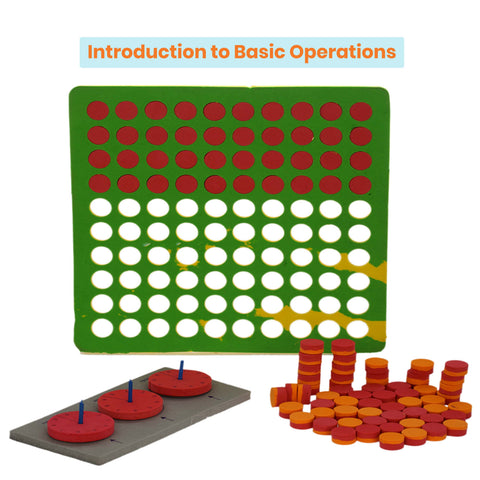Introduction to Basic Operations

Make an innovative tool to practice:

• Addition of three digit numbers with and without carry.
• Subtraction of three digit numbers with and without borrow.

Understand multiplication and repetitive addition through the math mat.

Understand division by grouping through the math mat.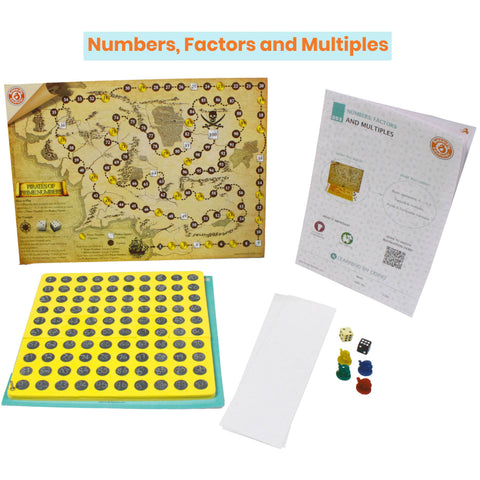Play and Learn Composite numbers, Perfect squares, Prime numbers, Factors multiples in a fun way using math board game

Use math wonder mat to find:

• Understand Factorization: Factors of a given number by dividing it into different number of groups.
• What are Multiples of a number? Learn through multiplication tables.
• Play the Pirates of Prime board game with dice to know composite numbers, perfect square, prime numbers in a fun way!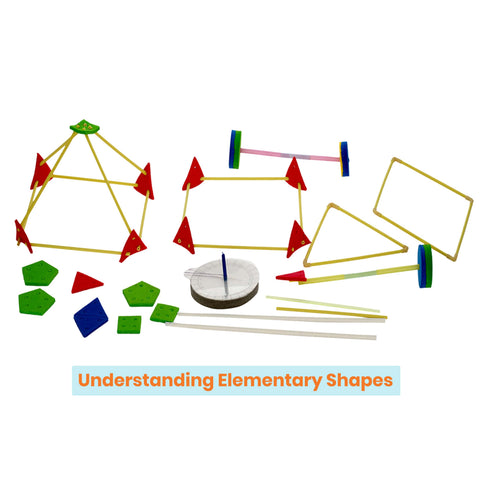Make shape of cuboid, shape of triangle, shape of cube | Make 2D shapes & 3D shapes to learn Elementary Shapes | Basics of Geometry Kit

• Make models to represent a ray, a line segment and a line & understand their differences
• Make a model to form different angles between line segments
• Make different 2D figures (triangle, square, rectangle) & find the no. of sides and vertices
• Make different 3D figures (cube, cuboid, pyramid) & find the no. of sides, vertices & faces
Sunday,Monday,Tuesday,Wednesday,Thursday,Friday,Saturday
January,February,March,April,May,June,July,August,September,October,November,December
Not enough items available. Only [max] left.